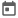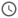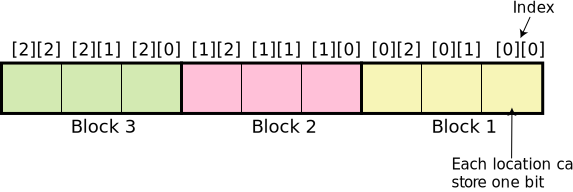# Introduction to Arrays in SV##### 21 Jan 2022##### 7 mins

In Verilog we have seen that only static arrays can be created. Static arrays has a major drawback as the size of the arrays once defined cannot be changed. This wasted a lot of memory space as at times the entire size of the array is not used. Dynamic arrays were introduced in system Verilog along with few other arrays constructs like associative arrays and queues. We will have a detailed look into the different kind of arrays constructs, but before that we will deep dive into the concept of packed and unpacked arrays. Knowing difference between packed and unpacked array is very crucial in SV.

### Packed Arrays

Packed arrays can be considered as a single dimensional memory, in which the index `i` represents the `ith` bit of the memory. Packed arrays can only be used with data types having width of one bit, i.e., only logic, bit, reg can be used. Any other data type cannot be used.

In the figure below, we see that all the data are closely packed with each address or index having one bit data. Thus, we cannot use multiple bits data type such as int, byte, etc to declare a packed array.

#### Declaration of Packed Arrays

For packed arrays, the square bracket `[ ]` comes before the identifier of the declaration. Inside `[ ]`, the size of each dimension is mentioned. This is same as Verilog. Index of MSB is mentioned first followed by colon and the index of LSB.

Syntax - `bit [<index of MSB>:<index of LSB>] <identifier>;`

##### Example
``````    bit [7:0] a;
bit [3:0] nibble;``````

#### Multi-dimensional Packed Arrays

In System Verilog, packed arrays can be of multiple dimensions. Multiple dimensions should not be confused with the physical layout. Physically it will be single dimension only. This concept can be visualised by the following figure.2-D Packed array of dimension [2:0][2:0]

So, multi-dimensional packed array just means that the single block of 1-D memory is split into different blocks inside the simulator. This can be beneficial when the size of packed array is large.

##### Example use case

Let us consider a situation in which we need to store 256 bits in a packed array. It would be difficult to keep tracks of each bit in binary format. Now this 256 bits can be split into 3-dimensional packed array with dimension `[2:0][2:0][7:0]`. Now it gets easy to initialize the data type. Also, it is more manageable. This is one use case, but there can be many more use cases.

#### Packed Array Slicing and Assignment

Array slicing is same as that in Verilog. We can use `+:`, `-:` and other slicing techniques as discussed in Verilog topic.

Assignment operation can be bit tricky in multi-dimensional packed array. Let us understand how to assign a value in multi-dimensional case using the example given in previous section. The example packed array is having 3 dimensions [3:0][3:0][15:0]. This array can be visualized as below figure.

From the image above, we can see that blocks can be selected in many ways to assignment that block. If we provide index in only the third square bracket, then level 3 location is selected as shown in figure. Thus, we need to give 64-bit value for each cell.
Ex – `arr[ ][ ] = 64’h456;` or `arr = 64’h8FF;` or `arr[2:1] = 128’h4557;`

Similarly, if we provide index in second and third bracket only, level 2 location will be selected. In this case we can assign maximum 16-bit of data as each level 2 location can store 16 bits.
Ex – `arr = 16’h2A;` or `arr[3:2] = 32’h453AA;`

Thus, if we provide all three indices, we can assign 1 bit of data which means we can assign a bit in any location of the array. This concept is very crucial as it is important while working with arrays and important for interviews as well.
Ex – `arr = 1’b1;` or `arr[10:0] = 11’b0110011;`

In Verilog, all arrays are of packed nature by default. Thus, there is no concept of packed & unpacked arrays in Verilog.

#### Example

``````module packed_arr_ex;
//  declaring 3-D packed array
//  total size - 256 bits
bit [3:0][3:0][15:0] arr;

initial begin
//  assignment with only one index
arr  = 64'h2548AA54;
arr [3:2] = 128'h458AAC445AD;

\$display("Assignment with only one index");
\$display("Arr = %h", arr);
\$display("Arr = %h", arr);
\$display("Arr[3:2] = %h\n", arr[3:2]);

//  assignment with 2 indices
arr  = 16'h12;
arr [3:2] = 16'h34;

\$display("Assignment with  2 indices");
\$display("Arr = %h", arr);
\$display("Arr = %h", arr);
\$display("Arr[3:2] = %h\n", arr[3:2]);

//  assignment with 3 indices
arr  = 1'b1;
arr [8:0] = 9'h4;

\$display("Assignment with  3 indices");
\$display("Arr = %h", arr);
\$display("Arr = %h", arr);
\$display("Arr[8:0] = %h", arr[8:0]);
end
endmodule``````
##### Output
``````# Assignment with only one index
# Arr = 000000000000000000000458aac445ad000000002548aa540000000000000000
# Arr = 000000002548aa54
# Arr[3:2] = 000000000000000000000458aac445ad
#
# Assignment with  2 indices
# Arr = 000000000000000000000458aac445ad000000002548aa540000003400000012
# Arr = 0012
# Arr[3:2] = 00000034
#
# Assignment with  3 indices
# Arr = 000000000000000000000458aac445ad000000002548aa540000003402040012
# Arr = 1
# Arr[8:0] = 004``````
Try this code in EDA Playground

### Unpacked Arrays

Unpacked arrays can be considered as a 2-dimensional memory. A 2 -dimensional memory means that each address of the memory can hold one or more than one bits of data, i.e., there is a width of memory. In simpler terms, it is the same type of array we are acquainted with in other programming language like C or Java. Unpacked arrays can be used with any data types, even 1bit data types.

In the figure below, we can see that each index can hold one or more bits of data and are not closely packed. The maximum location present in unpacked array is also known as the depth. In the figure below, the depth is 4 as there are 4 locations. The no of bits that can be stored in each location is known as width. These terminologies are mainly used in hardware memories but helps us understand the concept of unpacked array with ease.

#### Declaration of Unpacked arrays

For unpacked arrays, the square bracket `[ ]` comes after the identifier in the declaration. Inside `[ ]` the indices are mentioned. The first index is mentioned first followed by colon and then the last index. If the first index is 0, then array can be declared by just giving the number of locations in array. In this case, the last index will automatically be `no of location - 1`.

Syntax:

`<datatype> <identifier> [<first index>:<last index>];`
or
`<datatype> <identifier> [<no of locations>];`

Please not that in packed array we used MSB and LSB, as in packed array highest index is MSB, whereas lowest index is LSB

#### Multi-dimensional Unpacked array

Just as the packed array, unpacked arrays can also be of multiple dimensions. Again, physically it can be visualized as 2-dimensional matrix which is split in various blocks. The concept remains same as that of the packed arrays with slight difference as shown below.2-D Unpacked array of dimension [1:0][3:0]

So, it can be observed that the depth is split into different blocks by simulator. Please note that multi-dimensions are only a way of representation in the simulator for both packed and unpacked array.

#### Array slicing and Assignment

Array slicing is the same as that of the packed arrays.

While assigning unpacked arrays, we must keep in mind that each index will hold certain number of bits and the assignment should be made keeping in that mind.

We use loops or `‘{}` operator to assign elements to an unpacked array. Loops will be discussed later, thus that method will be explained in future articles. In this article we will focus on the operator method. `’{}` represents a element thus for arrays of multiple dimension, the `’{}` operator is nested within the same operator. This will be clear with the example mentioned below.

#### Example

``````module unpacked_arr_ex;
//  unpacked array declared using size of array
byte arr1;
//  unpacked array declared using mixed approch
byte arr2[3:0];

initial begin
arr1 = '{23, 32, 45, 20};
\$display("Arr1 is %p", arr1);

//  assignment with only one index
arr2  = '{8'h34, 12, 'hA, 'hF};
arr2  = 8'h67;
arr2 [3:2] = '{45, 78};

\$display("Arr2 is %p", arr2);

//  Multiple operator are nested for multi-dimensional
arr2 = '{'{34, 56, 87, 23}, '{12, 34, 57, 68}};
\$display("Arr2 is %p", arr2);
\$display("Arr2 is %p", arr2);
\$display("Arr2 is %p", arr2);
\$display("Arr2 is %p", arr2);
\$display("Arr2[3:2] is %p", arr2[3:2]);
end
endmodule``````
Please note that in format specifier `%p` is used. This is a special modifier which is used to display the entire unpacked array at once. By default, it prints all the element of the array in decimal number format. To display in another number format `\$displayh` or `\$displayb` can be used.
##### Output
``````# Arr1 is '{23, 32, 45, 20}
# Arr2 is '{'{45, 78, 0, 103}, '{52, 12, 10, 15}}
# Arr2 is '{'{34, 56, 87, 23}, '{12, 34, 57, 68}}
# Arr2 is '{34, 56, 87, 23}
# Arr2 is '{12, 34, 57, 68}
# Arr2 is 56
# Arr2[3:2] is '{12, 34}``````
Try this code in EDA Playground

### Mixed Arrays

Mixed arrays are the combination of packed and unpacked array. In most of the cases it will not be possible to use the inbuilt data types for array creation. In these cases, we can combine both the types in single array.

#### Example use case

Consider a scenario in which an array having 4 elements or locations needs to be declared and each element should store 128 bits of data. In this case we cannot use any inbuilt data type.

We can declare this array as shown below `bit [127:0] arr ;`

To make the array more manageable, we can convert the packed array part into multi-dimension. `bit [7:0][15:0] arr ;`

#### Array slicing and Assignment

Now, we have declared the array. But how to access the array for assigning values or reading the values. As we have used multi-dimensional packed array, there should be a way to access the blocks inside the array which makes it more manageable. So lets have a look how we can access different blocks of array continuing the same example. To access one element of 128 bit, we can use the unpacked array index
`arr  = 128'h234AD342;`

To access one block of 16 bits inside 1st element of the array
`arr  = 16'h23;`

To access one bit
`arr = 1'b1;`

#### Example

``````module mixed_array_ex;
//  declaring mixed array
bit [7:0][15:0] arr ;

initial begin
//  assignment with only one index
arr  = 128'h234AD342;
\$display("Assignment with only one index");
\$display("Arr = %h", arr);

arr  = 16'h23;
arr  = 1'b1;
\$display("Arr = %h", arr);
end
endmodule``````
##### Output
``````# Assignment with only one index
# Arr = 000000000000000000000000234ad342
# Arr = '{'{0000, 0000, 0000, 0000, 0000, 0002, 0023, 0000}, '{0000, 0000, 0000, 0000, 0000, 0000, 234a, d342}, '{0000, 0000, 0000, 0000, 0000, 0000, 0000, 0000}, '{0000, 0000, 0000, 0000, 0000, 0000, 0000, 0000}}``````
Try this code in EDA Playground
Please note the output can be slightly different in different simulator depending upon how the simulator treats the packed array. For example, in Questasim, the packed array is split into multidimensions while displaying but in Aldec Riveria, the packed arrays as showed as one entity. This can be seen by running the example in EDA playground given.